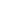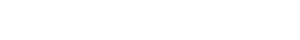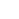# 103 people dead as Lassa fever spread to 16 StatesThe Nigeria Centre for Disease Control (NCDC) yesterday said 103 people have died of Lassa fever since its outbreak last December 30.
The figure is from 16 states, namely: Ondo, Edo, Ebonyi, Kano, Kogi, Kaduna, Taraba, Plateau, Bauchi, Enugu, Abia, Benue, Borno, Gombe, Sokoto and Katsina.
The number of confirmed cases this year stands at 586, NCDC said.
The report, released yesterday in Abuja, says Edo has the highest burden of confirmed cases at 35 per cent, followed by Ondo at 32 per cent, and Ebonyi at six per cent.
In total for this year, 26 states have recorded at least one confirmed case across 101 local government areas.
The report says: “Cumulatively from week one to week 07, 2020, 103 deaths have been reported with a case fatality rate (CFR) of 17.6 per cent which is lower than the CFR for the same period in 2019 (21.1 per cent).
“The predominant age group affected is 21-30 years (range: <1 07.="" 08023169485="" 08033565529="" 08052817243.="" 17="" 1:1.2.="" 2019.="" 33="" 63="" 78="" a="" abayomi="" according="" adds="" affected="" age:="" air="" akin="" all="" and="" any="" are="" arrival="" as="" at="" based="" bauchi="" beef="" been="" being="" biosecurity="" break="" but="" call="" came="" can="" carrying="" case="" cases="" centre="" circle="" citizens="" clinic="" collaboration="" commission="" commissioner="" compared="" confirmed="" contact="" containment="" determine="" develop="" did="" directorate="" disease.="" disease="" e="" early="" ebonyi.="" ebonyi="" emergency="" epidemiology="" explaining="" facility="" february="" federal="" female="" fever="" for="" force="" from="" further="" global="" government-approved="" government="" had="" has="" have="" he="" health="" healthcare="" hey="" him="" his="" hospital="" how="" href="https://thenationonlineng.net/103-die-of-lassa-fever-in-16-states/" identified="" idi-araba.="" if="" immediately="" improvement="" in="" increased="" index="" infected="" international="" is="" isolate="" isolated.="" isolation="" katsina="" lagos="" lassa="" law="" line="" lines:="" luth.="" luth="" malaria="" male="" man="" managed="" may="" median="" ministry="" monitored.="" ncdc="" nearest="" new="" nigeria="" nigerian="" no="" non-response="" not="" number="" of="" on="" onikan="" operation="" or="" origin="" other="" our="" out="" outbreak="" patient="" people="" period="" pick="" point="" positive="" prof.="" programme="" ratio="" recover.="" referred="" report="" reported="" reporting="" said.="" said="" same="" saying="" school="" significantly="" since="" so="" spread="" standard="" standards="" state="" statement="" states="" stem="" strategies="" surveillance="" suspected="" symptom="" target="_blank" teaching="" test="" that="" the="" them="" then="" there="" they="" those="" through="" to="" tracing="" transferred="" transmission="" treated="" treatment="" two="" typhoid.="" university="" unwell.="" up="" urged="" ward="" was="" we="" week="" well="" were="" when="" where="" while="" who="" will="" with="" workers="" working="" years="" yesterday="">Source 1>

Share this post with your Friends onNo Responses Yet

NOTE:- Make your comment a bit long to get it approved.

{{#message}}{{{message}}}{{/message}}{{^message}}Your submission failed. The server responded with {{status_text}} (code {{status_code}}). Please contact the developer of this form processor to improve this message. Learn More{{/message}}

{{#message}}{{{message}}}{{/message}}{{^message}}It appears your submission was successful. Even though the server responded OK, it is possible the submission was not processed. Please contact the developer of this form processor to improve this message. Learn More{{/message}}

Submitting…

FL MUSIC OF THE WEEKFL VIDEO OF THE WEEK

Looking for something? Search belowCHECK OUT OUR INITIATIVES